## The Geometry of the Cubic Formula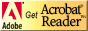This page is based on: R.W.D. Nickalls, A new approach to solving the cubic: Cardan's solution revealed, Mathematical Gazette 77 (1993), 354-359. You can download this article as a PDF (Acrobat) file.

Note that this page will use a little bit of calculus, but you can read (and hopefully understand) most of it without knowing calculus.

#### Depressing the cubic equation.

Once again we consider the cubic equation

ax3+bx2+cx+d=0.

Tartaglia's first step was to depress the cubic by shifting the graph of the cubic horizontally by the quantity b/3a. What does this mean for the roots of the cubic? The sum of the roots of the depressed cubic (counted algebraically) becomes 0:

Let the roots be denoted by x1,x2 and x3. The cubic then has the form

a(x-x1)(x-x2)(x-x3).

Multiplying out we obtain:

ax3-a(x1+x2+x3)x2+a(x1 x2+x1 x3+x2 x3)x-a x1 x2 x3.

Thus setting b=0 (depressing the cubic) means x1+x2+x3=0, and vice versa.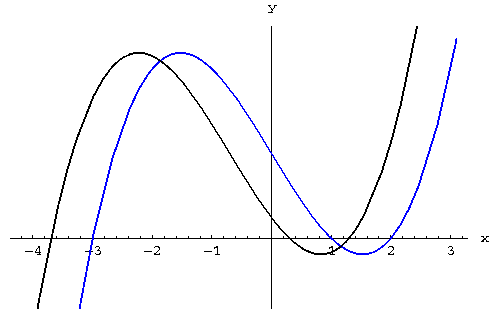A cubic (in black) and its depressed counter part (in blue). Note that the roots of the depressed cubic add up to 0.

#### The "easy cubic".

By shifting the graph of a depressed cubic down by the quantity d, we can eliminate the constant term of the equation; such a cubic will have the form

f(x)=ax3+cx.

Note that this cubic is odd. Since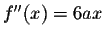(and its third derivative is not 0), it will have its only inflection point at the origin. What can we say about the relative extrema? If it has any, it will have one local minimum and one local maximum: Since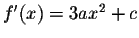, the extrema will be located at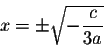This quantity will play a major role in what follows, we set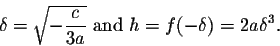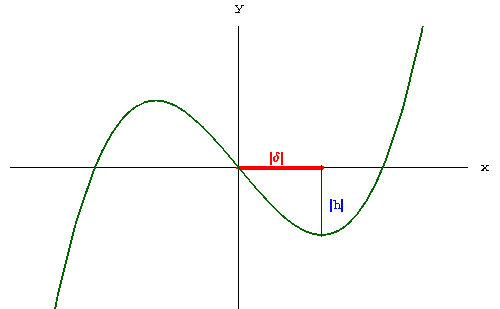The quantity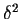tells us how many extrema the cubic will have: If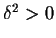, the cubic has one local minimum and one local maximum, if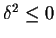, the cubic has no extrema.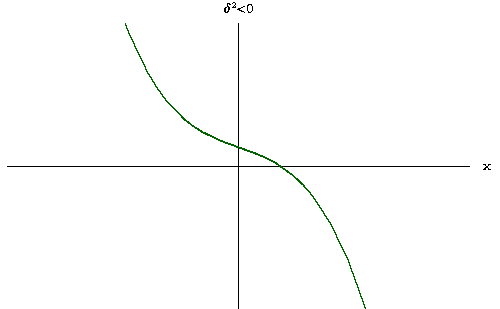#### Back to the "general cubic".

Let's go back to the general cubic

f(x)=ax3+bx2+cx+d.

The meaning of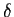and h has not changed; I leave it to you to check that in terms of the four coefficients a,b,c,d: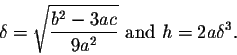Here comes the most crucial observation of this page: It is now obvious to see that the cubic will have one real root when |d|>|h|, or equivalently if d2>h2. If, on the other hand, d2<h2, the cubic equation will have 3 real solutions. We will see later (and that is easy), what happens when d2=h2.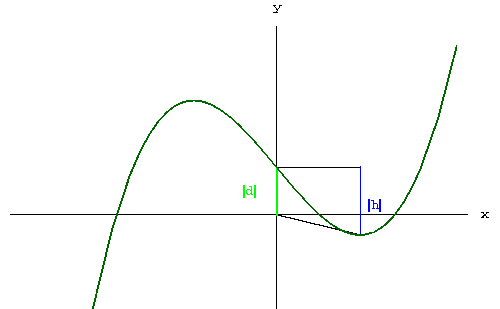The relative size of |d| and |h| determines the number of real roots.

Let's now follow Cardano's approach and see where the quantity d2-h2 enters the picture.

We want to solve an equation of the form

ax3+bx2+cx+d *=0.

Using Tartaglia's substitution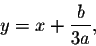we obtain the equation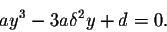Here d is a quantity depending on a, b, c, and d *.

Using the notation on the "Cubic Formula" page,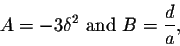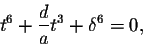which yields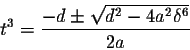Remembering that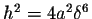, we can write this as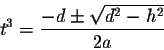There it is: d2-h2!

#### The case d2-h2>0: One real root.

Let's look at the example

x3-2x-4=0.

(No secrets: You can check that the roots are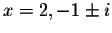.) In this case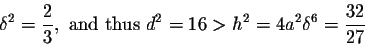Cardano's approach yields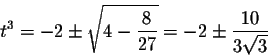Discarding the solution with the negative sign we obtain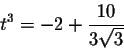Now it helps tremendously to notice that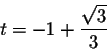Indeed, you can check that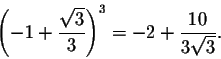(Note that this is cheating, though; there is no purely algebraic way to figure this out: Try it!). Consequently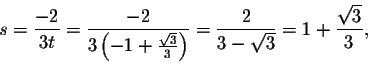and finally the real root is given by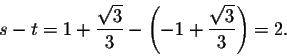Remark: I have presented an example, where. If, on the other hand,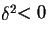, the cubic function will have no extreme points, and thus exactly one real root. You can check that the algebra still works the same if: We are never usingor h in our calculations, onlyand h2. Thus all calculations will still only deal with real numbers.

#### The case d2-h2<0: Three real roots.

Let's look at the example

x3-7x+6=0.

(No secrets again: You can check that the roots are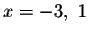, and 2.)

Our goal is to find one real root; the other two real roots can then be found by polynomial division and the quadratic formula.

In this case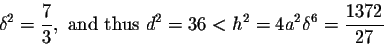Cardano's approach yields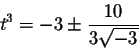I hope you are not surprised by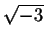; we were expecting that! Consequently, discarding the solution with the minus sign, we obtain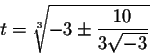Thus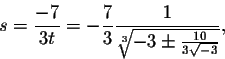and one of the solutions to the cubic is given by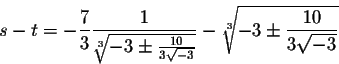A paradox: Even though the solution is real, Cardano's formula contains imaginary numbers. Cardano and his colleagues called this the Casus Irreducibilis.

The Casus Irreducibilis historically led to the study of complex numbers. You might be surprised that complex numbers did not enter the picture via the quadratic formula, as they usually do nowadays in the school curriculum. The reason: There was no "paradox"! The complex solutions could not be seen, they were "imaginary".

One of the great algebraists of the 20th century, B.L. van der Waerden observes in his book Algebra I, that the Casus Irreducibilis is unavoidable. There will never be an algebraic improvement of the cubic formula, which avoids the usage of complex numbers.

#### Using a Trigonometric Substitution to Solve the Casus Irreducibilis.

We will now violate the spirit of Cardano's computations by using transcendental functions to find the roots of a polynomial.

Let's consider the depressed cubic equation

ax3+cx+d=0

again. We will use the trigonometric substitution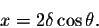Substituting in the cubic yields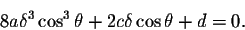Using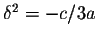, we can write this as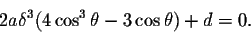Next observe that by deMoivre's formula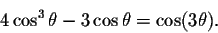Finally remembering that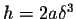, it follows that we can write the cubic equation as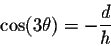We will only obtain solutions for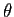, if the right hand side is bounded by 1 in absolute value, i.e., if

d2<h2.

Thus this method will only work when the cubic has three real roots, in the Casus Irreducibilis.

In this case,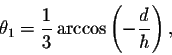is one solution of the trigonometric equation, yielding as a solution to the cubic equation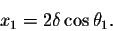It is not hard to see that the other two solutions are given by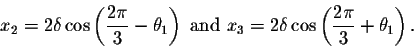Let's try this for our example

x3-7x+6=0.

Here,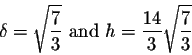Consequently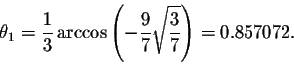This yields the solutions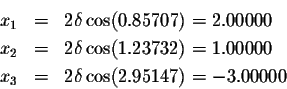#### The case d2-h2=0: Repeated roots.

I will assume that d=h, leaving the case d=-h to you. Using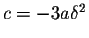and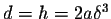, we can rewrite

ax3+cx+d=0

as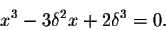By "inspection", we can see that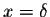is a root of this polynomial; in fact, the polynomial has an elementary factorization: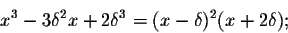Thus the cubic equation has the roots,and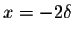.[Back: The Cubic Formula] [Next: The Quartic Formula]
[Algebra] [Trigonometry] [Complex Variables]
[Calculus] [Differential Equations] [Matrix Algebra]S.O.S MATHematics home page

Do you need more help? Please post your question on our S.O.S. Mathematics CyberBoard.Helmut Knaust